## A 0.060-kg ice hockey puck comes toward a player with a high speed. The player hits it directly back softly with an average force of 1.50 x

Question

A 0.060-kg ice hockey puck comes toward a player with a high speed. The player hits it directly back softly with an average force of 1.50 x 10^3 N. The hockey stick is in contact with the ball for 1.20 ms, and the ball leaves the stick with a velocity of 8.00 m/s. Let the direction of the force be the + x direction. Find the following (note: be careful with the sign/direction of the values):_______. 1. The final momentum of the ball 2. The impulse on the ball 3. The initial velocity of the ball

in progress 0
6 months 2021-07-20T02:52:33+00:00 1 Answers 4 views 0

Explanation:

Given

mass of puck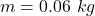Average force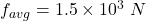time of contact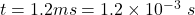puck leaves with a velocity of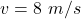We know impulse is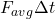$$=\text{change in momentum}$$

therefore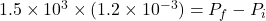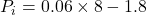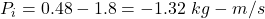Final momentum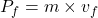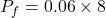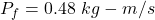Impulse on the ball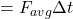Impulse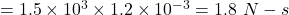Initial velocity is given by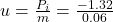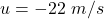i.e. initially ball is moving towards -x-axis